# 15 EXACT Math Skills Required To Pass IB Physics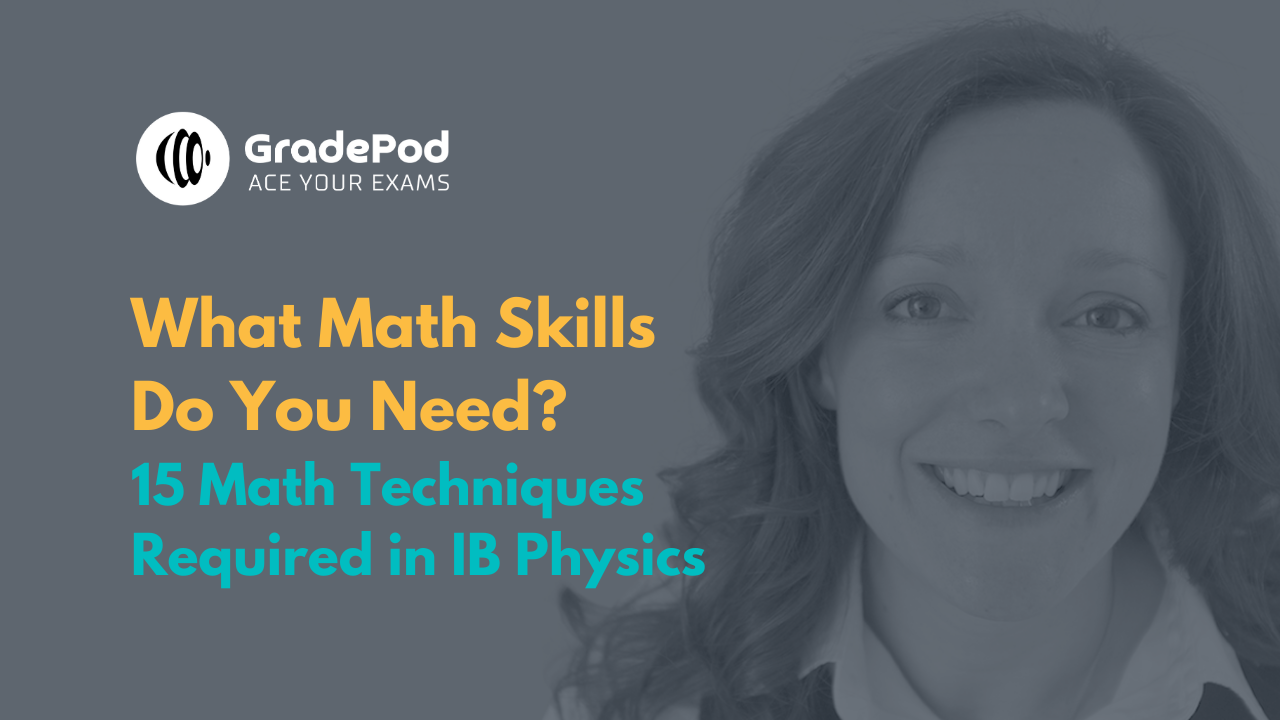### I've lost count of the amount of time I have said this to my students...

“It is super important that you understand Maths if you are going to progress in IB Physics. The two subjects compliment each other perfectly and mathematical techniques are vital in solving physics problems.”

The trouble is....

I know you have a lot of questions about what maths EXACTLY is included in IB Physics.

#### Let me tell you the 15 maths skills required in IB Physics:

1. Perform the basic arithmetic functions: addition, subtraction, multiplication and division
2. Carry out calculations involving: means, decimals, fractions, percentages, ratios, approximations and reciprocals
3. Carry out manipulations with trigonometric functions
4. Carry out manipulations with logarithmic and exponential functions (HL ONLY)
5. Use standard notation
6. Use direct and inverse proportion
7. Solve simple algebraic equations
8. Solve linear simultaneous equations
9. Plot graphs: including two variables that show linear and non-linear relationships
10. Interpret graphs: including significance of gradients, intercepts and areas
11. Draw lines of best fit on scatter plot graphs
12. Construct linear lines of maximum and minimum gradients (and error bars)
13. Interpret data in various forms: bar charts, histograms and pie charts
14. Represent the arithmetic mean
15. Express uncertainties to 1 or 2 significant figures

If you'd like a full explanation behind these math skills:

#### But first...

I know that you have more questions.

#### Does IB Physics require a lot of maths?

Yes and no! Maths and Physics go together like fish and chips! If you'd like a career that involves Physics, then Maths is an essential skill that you should embrace. However, if you simply need to pass the IB Physics exam with little interest in Maths then there are very specific skills that you need to master for the final exams and they can be learned with practice and determination.

You can watch this video covering: How to Solve IB Physics Exam Questions involving Math

#### Is IB Physics HL calculus based?

No. You do not need to use calculus in IB Physics HL. There are parts of the course where calculus fits in perfectly, namely Simple Harmonic Motion (Topics 4&9) and Equations of Motion (Topic 2) but the specification does not dictate that calculus should be used.

#### Can I still study IB Physics (SL or HL) with Maths Studies?

I have taught many students in both HL and SL Physics who have achieved fantastic grades in IB Physics whilst studying Math Studies (instead of SL and HL Maths). Your school may have a policy on which Maths course you should take when choosing IB Physics, but if you are prepared to work hard then there shouldn't be any barriers for you to achieve a 7 in IB Physics whilst studying Math Studies.

Here is Felicia, who studied HL Physics with me and did Math Studies at the same time: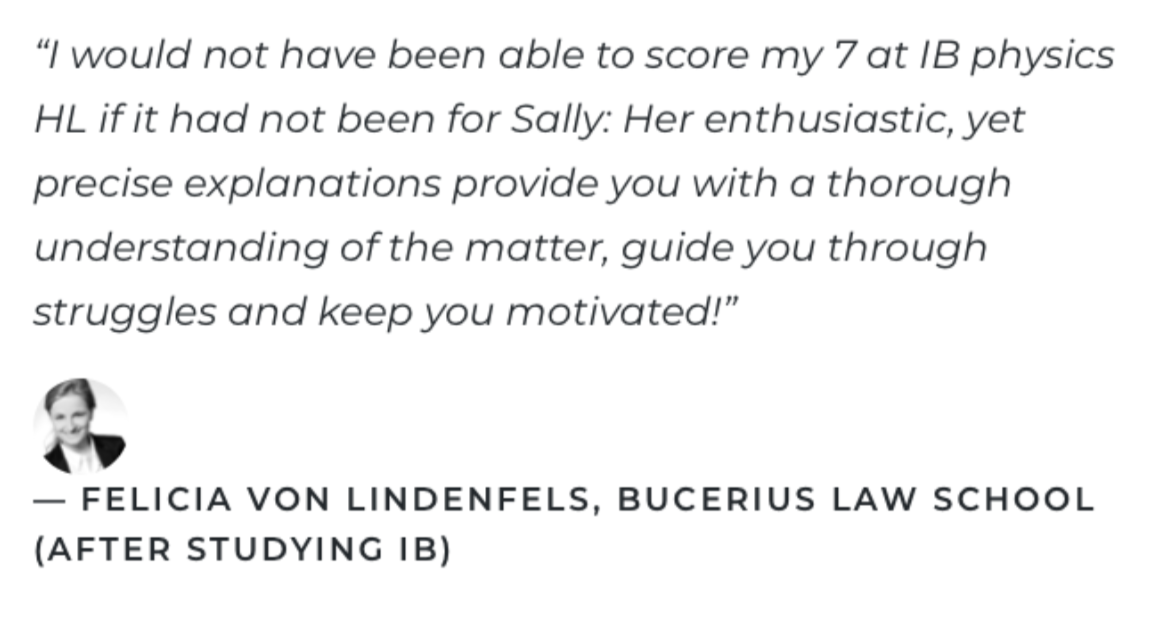Just remember... if you'd like a career that involves Physics, then Maths is an essential skill that you should embrace.

### 15 Math Skills Needed in IB Physics [EXPANDED VERSION]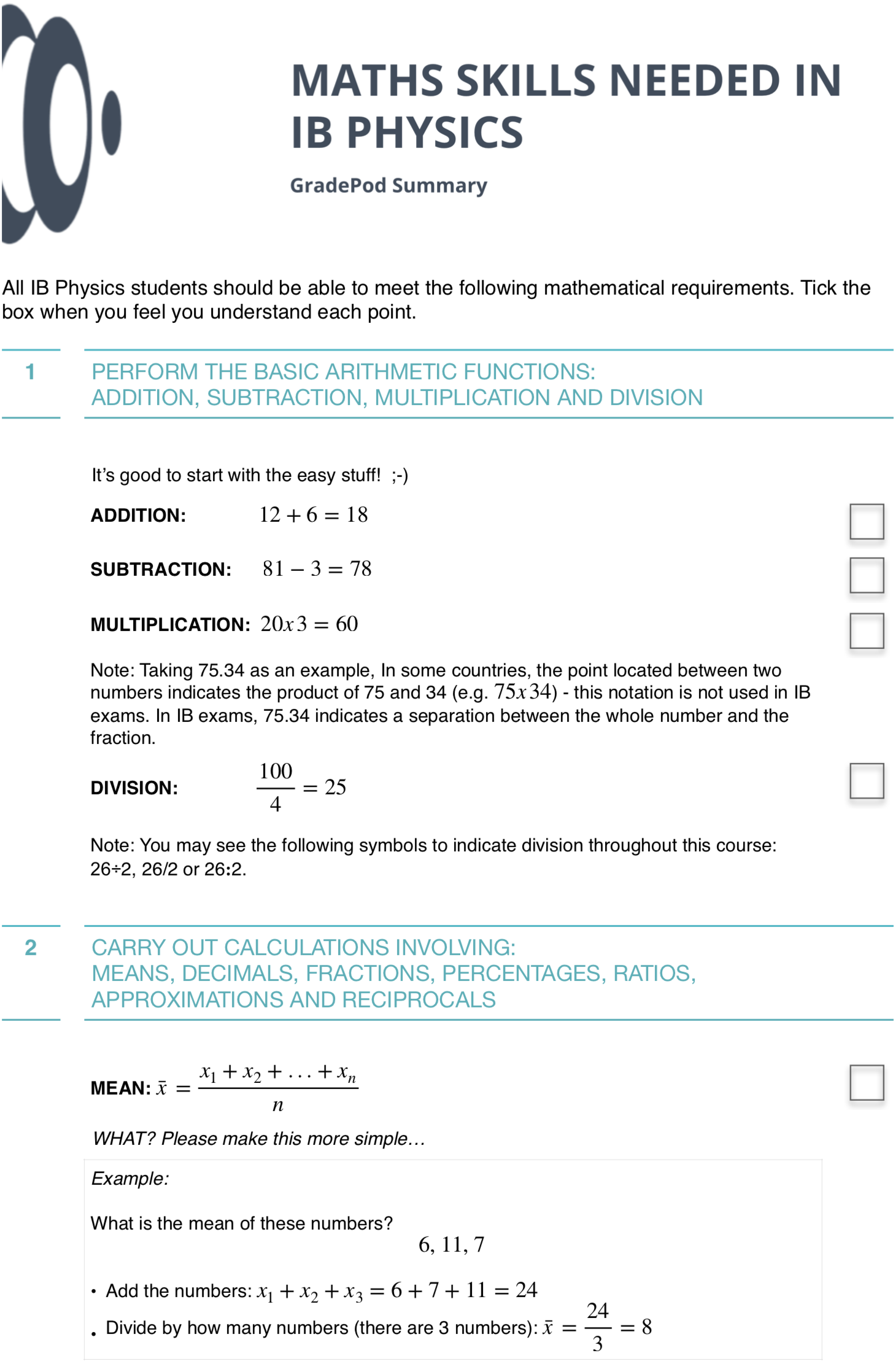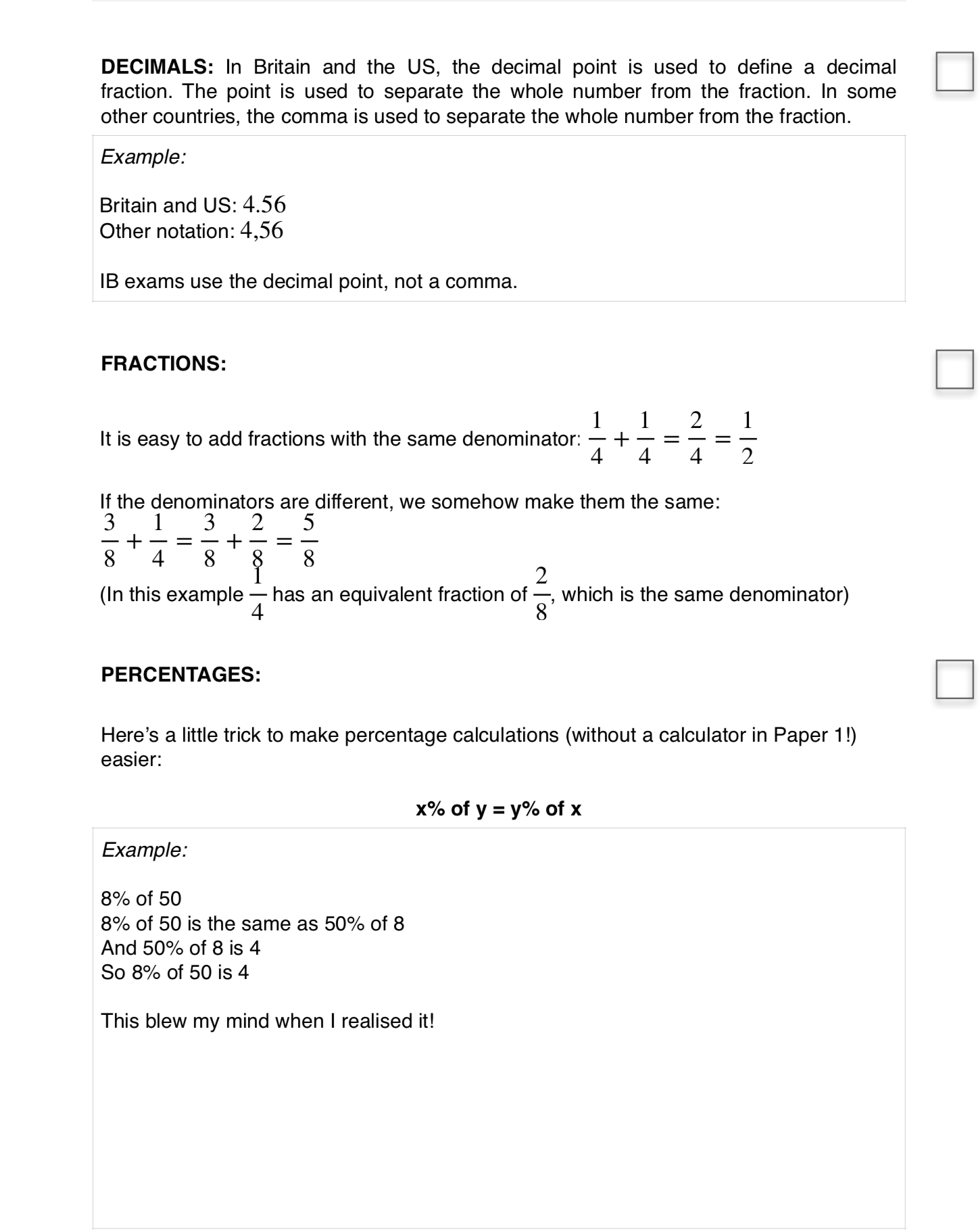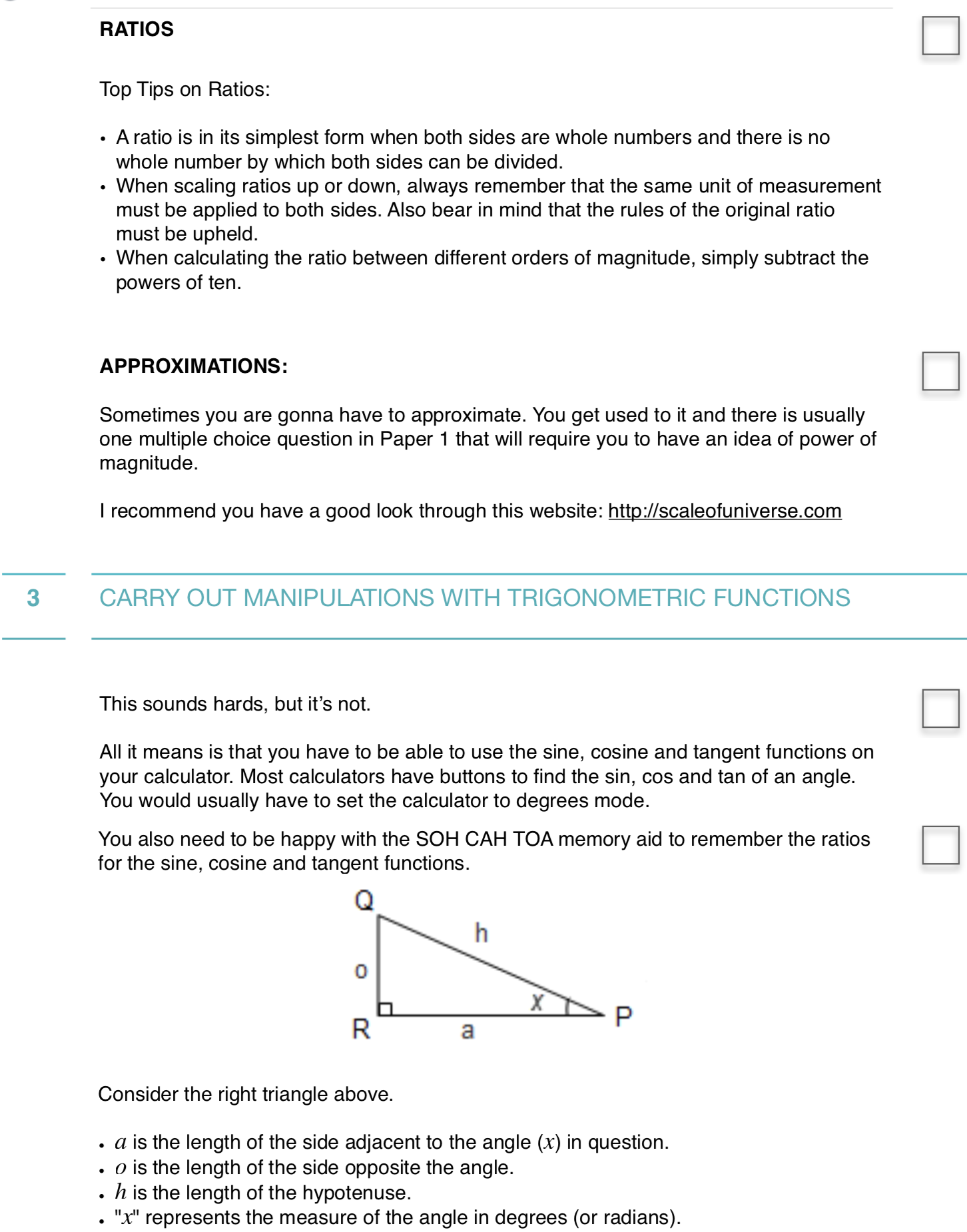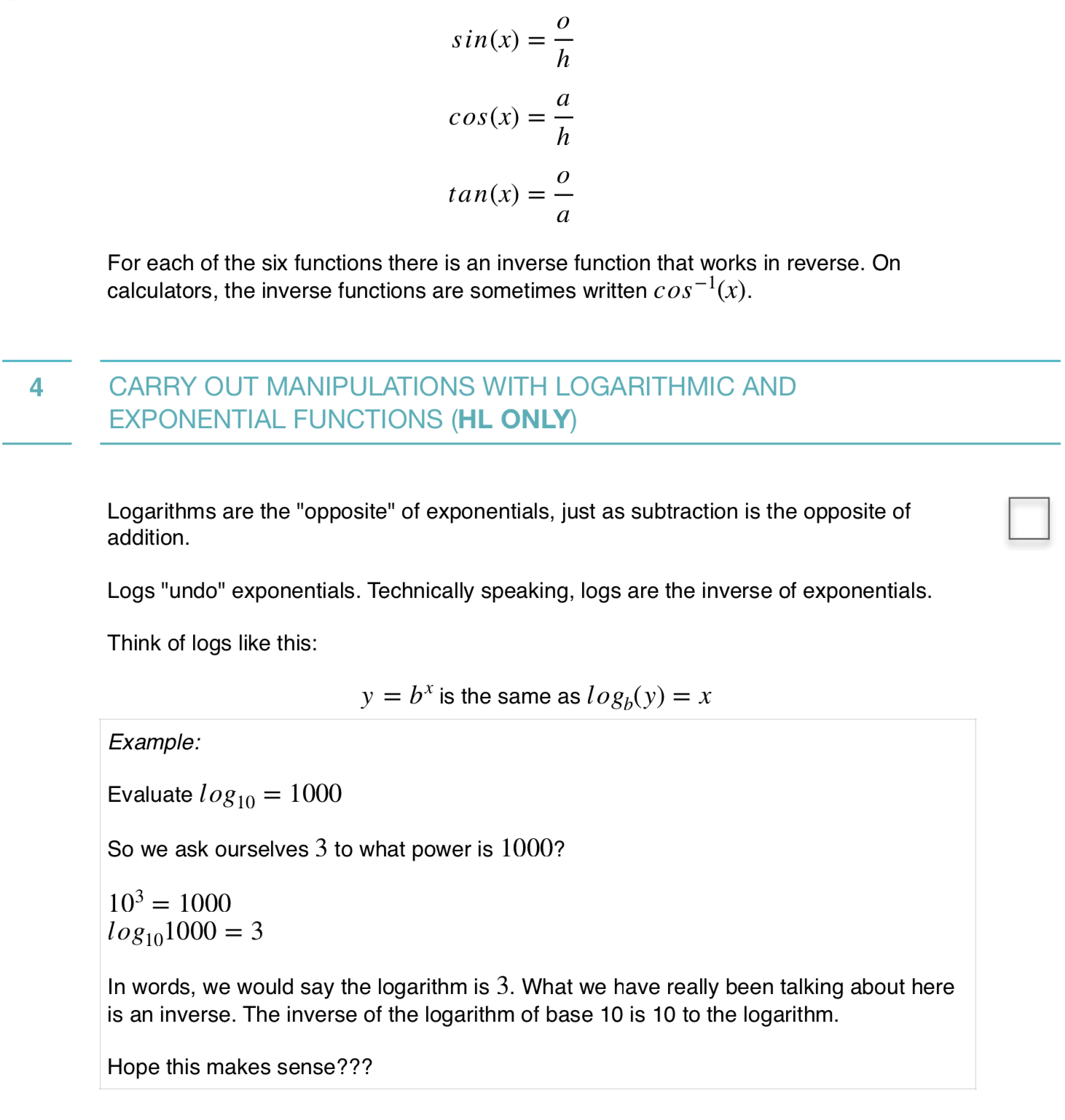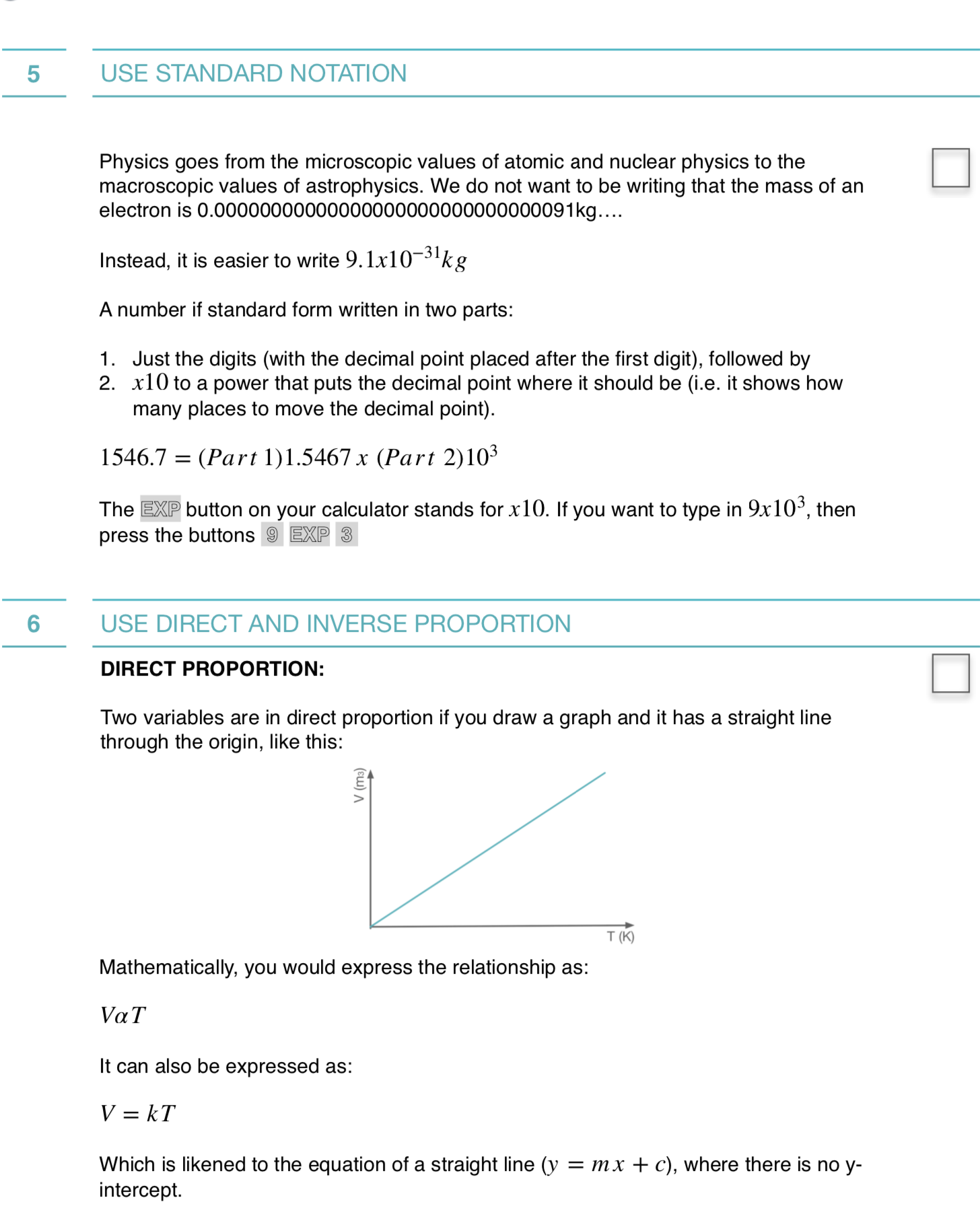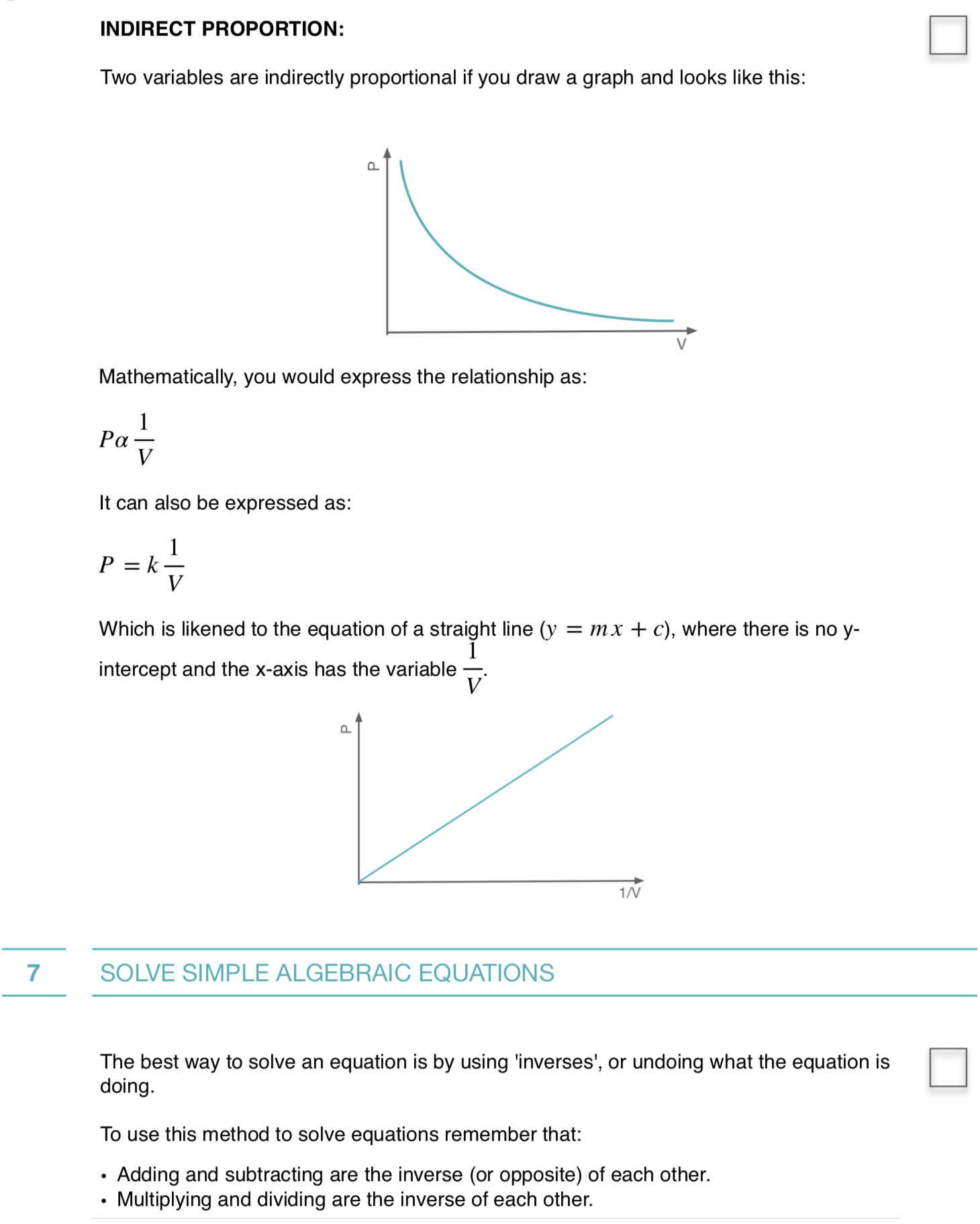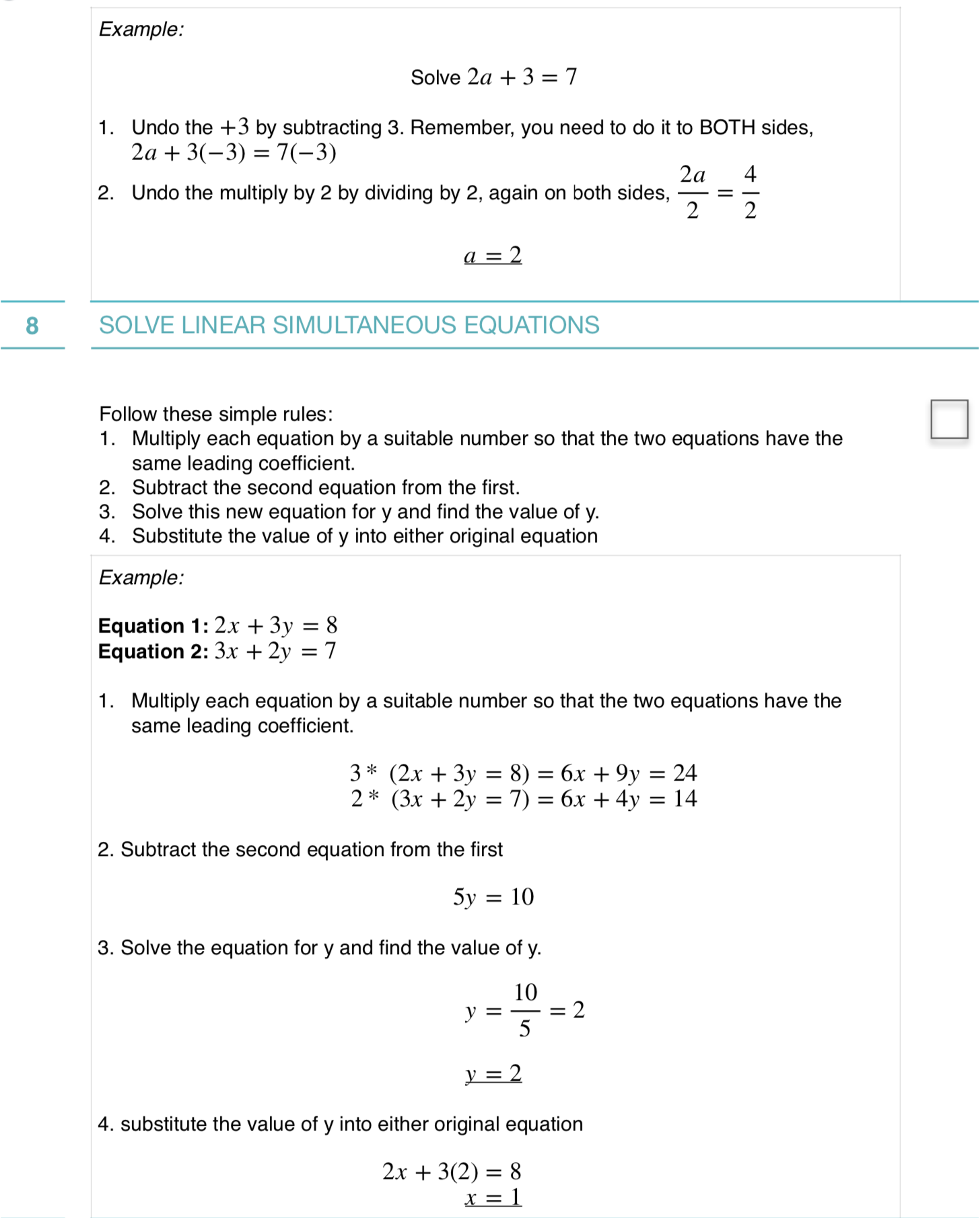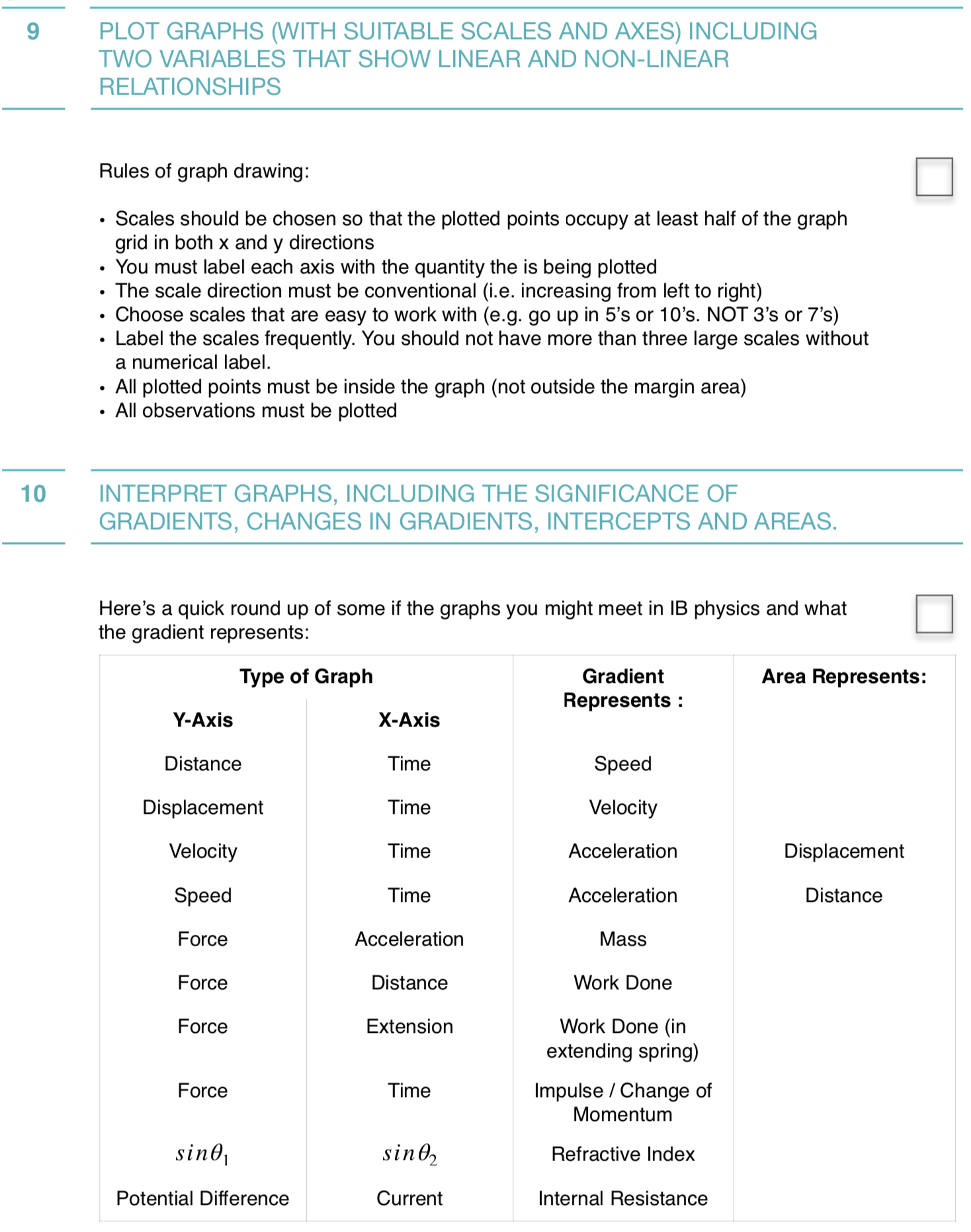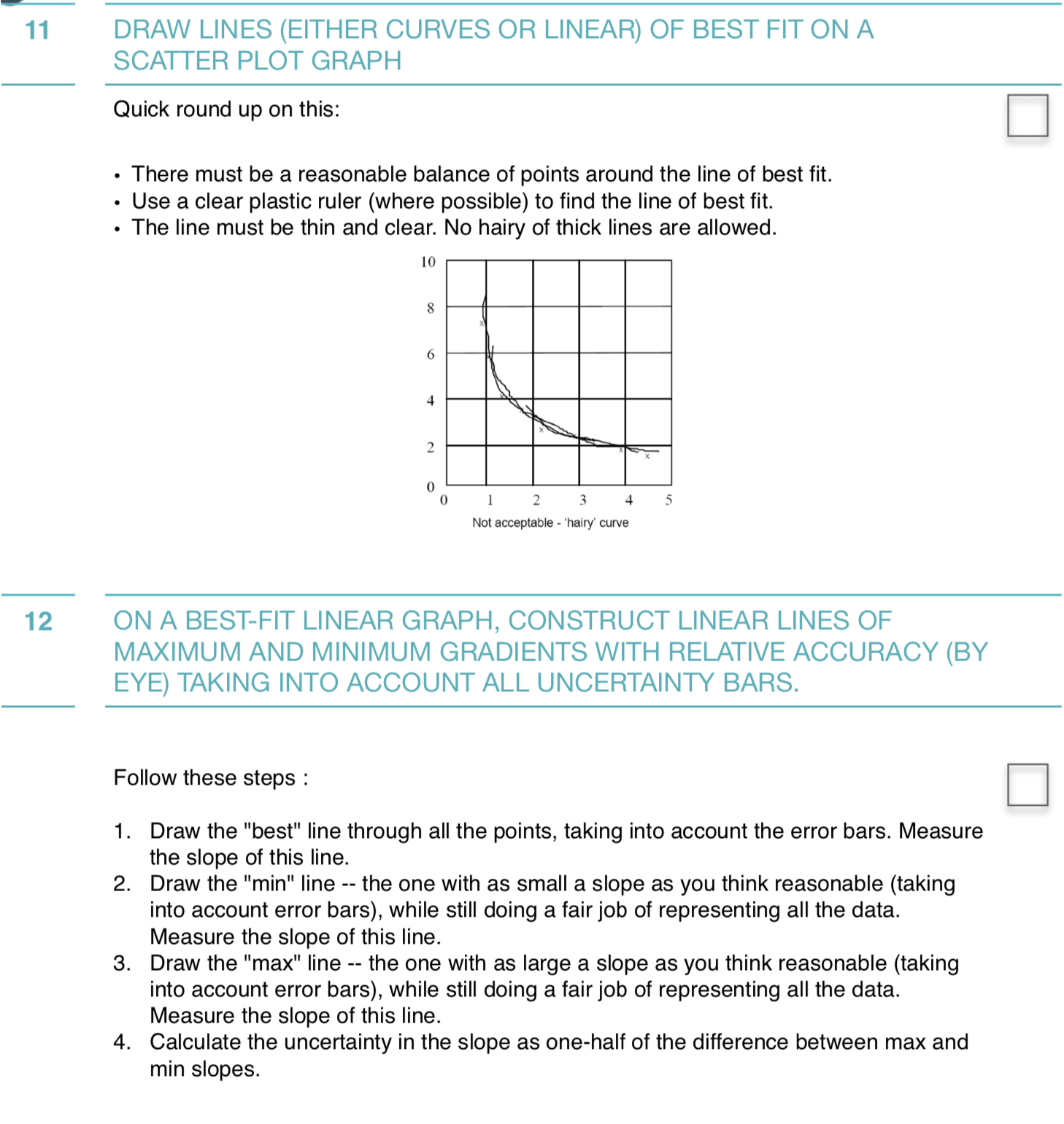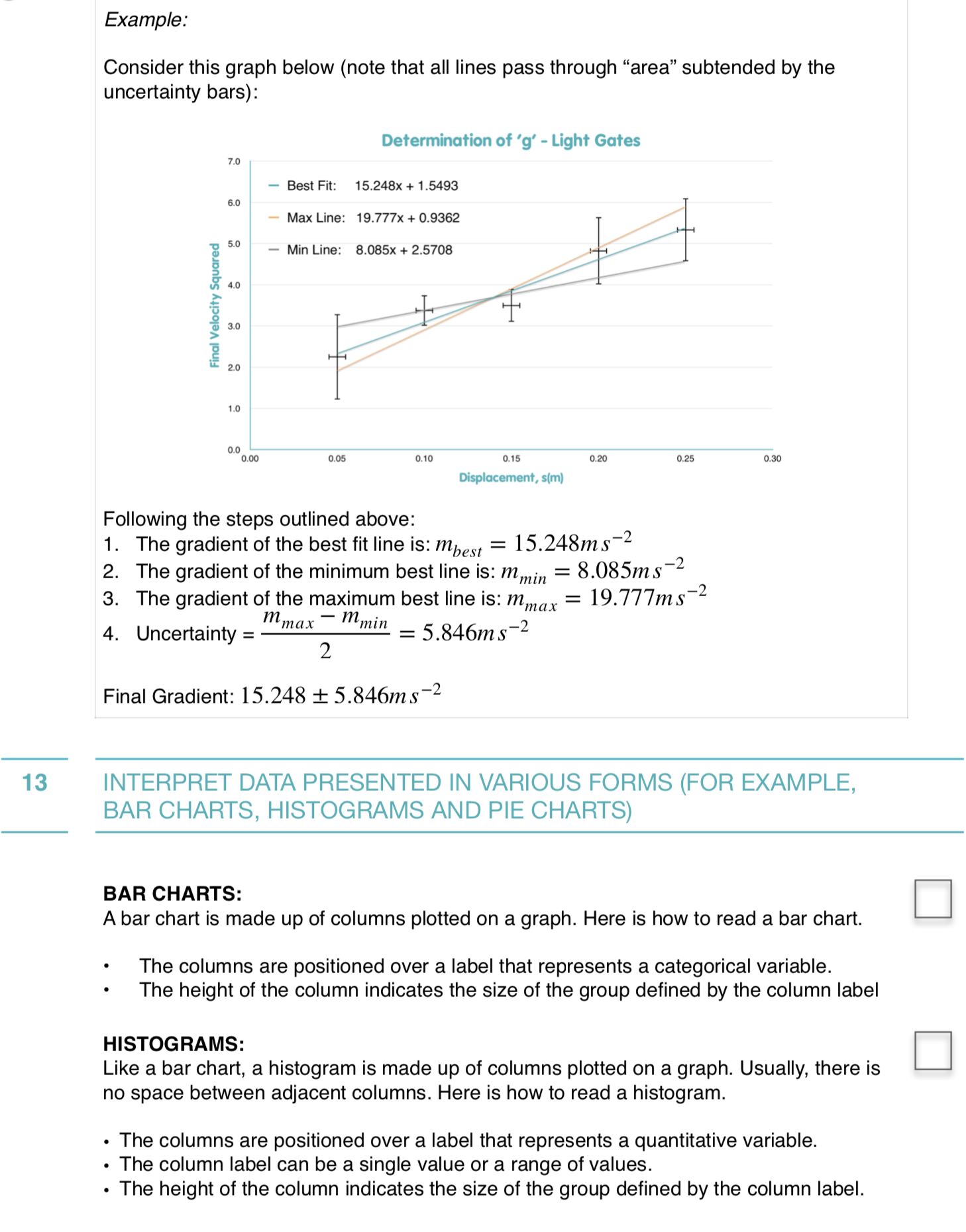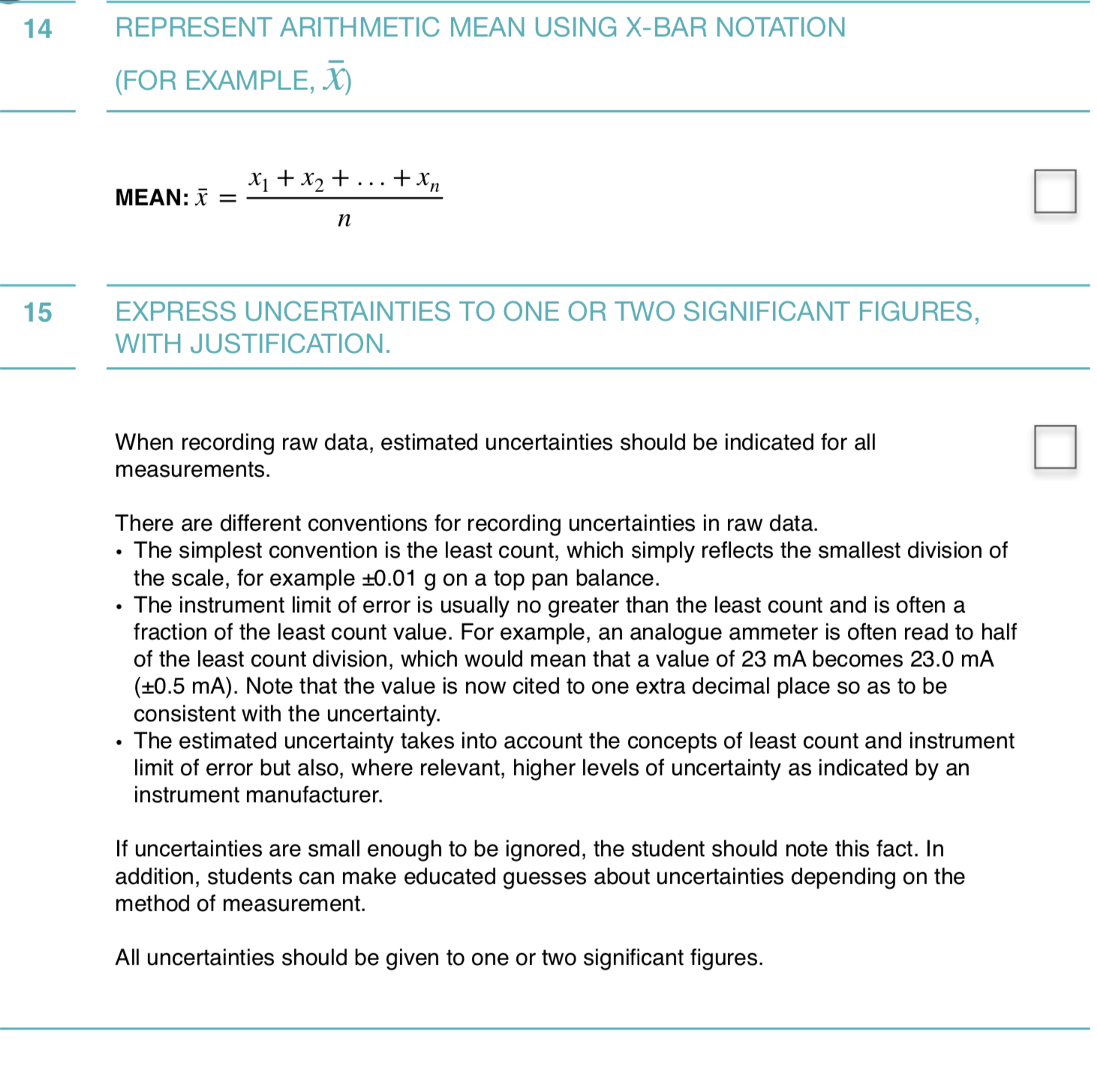Hope this helps### FREE eBook: The ULTIMATE IB Physics Internal Assessment Guide